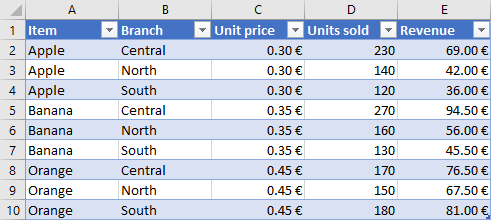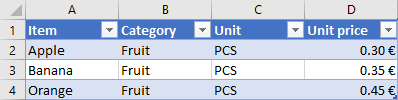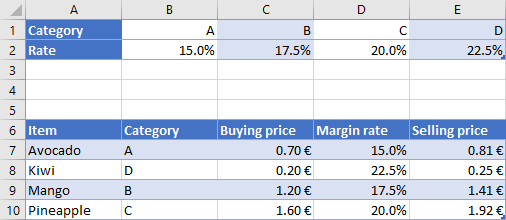﻿ Excel functions VLOOKUP and HLOOKUP - formula examples | AnswerTabs

# Excel functions VLOOKUP and HLOOKUP - formula examples

Would you please provide working examples of VLOOKUP and HLOOKUP functions ? The formulas I have created are not working for some reason.
Hi,

VLOOKUP function in the following example is used to get the unit price from the second table, that is created on another sheet.

The first table:The second table on another sheet:To get unit price from the second table for C2, the formula should look like:

=VLOOKUP(A2;Sheet2!\$A\$2:\$D\$4;4;FALSE)

A2 - lookup value
Sheet2!\$A\$2:\$D\$4 - table array, which describes the fixed location of the second table
4 - the column index number, which describes the column where the value is located
FALSE - used to apply the exact match

When you are using the tables as objects, the formula in C2 can also look like:

=VLOOKUP([@Item],Table2,4,FALSE)

HLOOKUP function in the following example is used to get the percentage rate from the first table, to calculate the selling price:To get percentage rate from the first table for D7, the formula should look like:

=HLOOKUP(B7;\$B\$1:\$E\$2;2;FALSE)

B7 - lookup value
\$B\$1:\$E\$2 - table array, which describes the fixed location of the first table
2 - the row index number, which describes the row where the value is located
FALSE - used to apply the exact match
Related questions